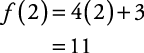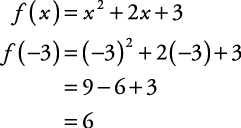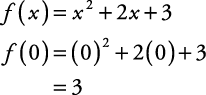## Function Notation

An equation involving x and y, which is also a function, can be written in the form y = “some expression involving x”; that is, y = f ( x). This last expression is read as “ y equals f of x” and means that y is a function of x. This concept also may be thought of as a machine into which inputs are fed and from which outputs are expelled. For each domain value input, there is a range value output.

If y = 4 x + 3, then f ( x) = 4 x + 3. The question “What is y when x = 2?” would be expressed as “What is f (2)?” if

f ( x) = 4 x + 3

thenThe statement f (2) = 11 says, “When x equals 2, y equals 11”; that is, the ordered pair (2, 11) belongs to this function. That also may be read as “when a 2 is input into this function, an 11 is output.”

##### Example 1

If f ( x) = x 2 + 2 x + 3, find each of the following.

1. f (–3)

2. f (0)

1.2.